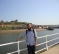## Resolution vs Sampling SpeedStarted by 5 years ago8 replieslatest reply 5 years ago2828 views

Hello:

I'm asking this as a bit of a newbie (do embedded prg as well) and did not take DSP at secondary level, and have some exposure to it. I know there's DSP functions in the CMSIS library which I have experimented with. Here's the questions.

Q1 - If I am sampling a signal that is 100Hz, and want to get 1Hz resolution, is it possible to sample at (for example) a 1KHz rate, and run DSP on it multiple times in one second, let's say 10? If not, what would the maximum be?

Q2 - If I sample at rate that isn't a base 2 multiple of the FFT block size, such as using DMA (not timer triggered) how will I know what bin the frequency of interest ends up in? (recall I am using the CMSIS FFT calls, i.e., arm_cfft_f32)

Thanks....Gary

[ - ]Your specification of the problem isn't exactly clear to me, but I'll give an answer to what I think you mean.

Q1: Whatever you want to do with your signal afterwards you have to make sure, your sampling rate is bigger than two times your maximum frequency. In practice - make it a lot bigger. 1kHz for a 100Hz signal should be OK, you have to apply an analog low-pass filter that cuts signals >500Hz at the input though - otherwise, your digital signal will get distorted. A first order analog low pass with 100Hz cut-off frequency would attenuate frequencies above 500Hz by a little more than 12dB which is rather bad, but if your input fdoes not contain higher frequencies in the first place that should be enough.

Sampling theory
Nyquist rate
Aliasing

Q2: Once you have sampled your data at rate Fs, you can perform ffts on it. The frequency each fft-bin represents is a function of the sample-rate and the block-size. Your first bin represents 0Hz. The distance between bins (resolution) is the rate divided by the block size.

If you collect 128 samples at a sampling rate of 1kHz for example, your first bin would be representing 0Hz, the second 1kHz/128=7.8Hz, the third 2*1kHz/128=15.6Hz and so on.

If you want an fft-resolution of 1Hz you would have to either increase the block size or decrease the sample-rate. @Fs=1kHz, a block size of 1024 samples will result in a resultion of less than 1Hz.

[ - ]If I understand correctly from your answer, it is not possible to get 1Hz resolution in less than 1 second time frame, no matter what sample rate and block size?

[ - ]well, you could collect only 128 samples and fill the rest with zeros - your fft will have a resolution of less than 1Hz then, but also the spectrum of your signal will be 'smeared', so yes and no...

If you just want to analyse your signal repeatedly, you could also take a buffer of 1024 samples, drop the oldest 128, add the newest 128 and perform a 1024 bin FFT - and you could do that every 128 samples...

[ - ]Hi,

Thanks for the clarification. Yes, correct - I do want to run it continuously.

Is this what is called overlap and add?

If I remove sample x to x, and shift all samples up by 128, does new sample get added to the "tail" of the array? ( x[1024-128] )

Does this method hold true then with a larger block, and increase processing speed, for example, 256?

[ - ]Yes, new samples are added to the tail.

If you only want to monitor the signal (no changes made), this method will work just like that.

If you want to alter the signal, you could kind of do this and it would be overlap and add, but you would need to window your signal first, then tranform and change, tranform back (maybe window again) and add to the overlapping old buffers. That would be a lot more complicated.

You might want to look up "fast convolution", "block convolution" and "overlap and add".

[ - ]I've tried to find a book with some practical examples of DSP in C, specifically overlap and add, but the problem of course is not knowing all the detail of what the content is.

[ - ]To answer your first question, the resolution is generally related with the FFT bins number. Before that, the sampling rate should be at least twice the maximum frequency in your signal. In acoustics it's usually 4 times. So let us assume that you same at 400 Hz. To get a resolution of 1Hz you should use 400 bins FFT. It is better to have the number of bins to be a power of 2 for computational complexity purposes. So I suggest to use 512-bins FFT, which gives you around 0.78Hz of resolution.

[ - ]